Intro to Programming: For Loops

In this article in my Intro to Programming series, we're going to talk all about for loops.
By Ciprian Stratulat • Jan 11, 2022

For Loops

In this article, we're going to start talking about another control flow structure. Namely, the for loop.

In previous articles, we started talking about control flow. In general, control flow refers to the order in which lines in a computer program get executed, as well as how many times each line of code gets executed. It might be a bit of a strange term if you haven't come across it before, but perhaps it will help if you think about it this way: control refers to computer code, because computer code controls what the computer does. Flow is how this computer code is executed. Similar to a river that flows and may have different incoming streams or ramifications, if-statements allow us to specify branches in our computer code.

To continue with the river analogy, rivers may also occasionally have whirlpools, areas where the water simply swirls in circles until it escapes the whirlpool and continues its normal flow.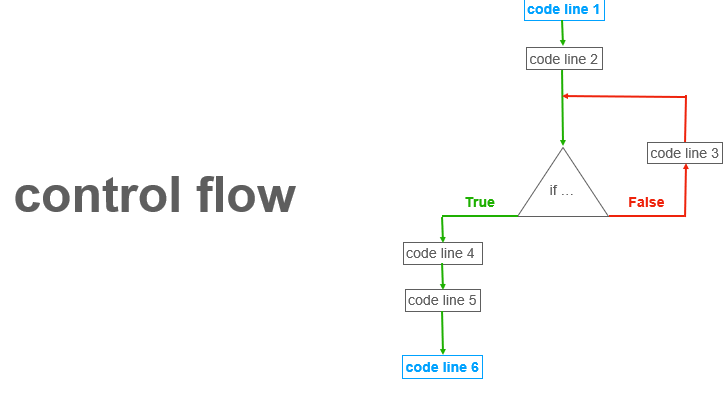I made a small diagram to reiterate this point. This is called a “logical flow diagram”. You can think of each box here as a line of code that makes up some program. It doesn't matter what the program does, we're only looking at the order in which the lines get executed.

The code normally flows in a straight line from top to bottom, following the green arrows, starting with the first line at the top - the blue box. Code line 1 is followed by code line 2. But at some point, we encounter an if statement, some condition that forks our execution. If the condition is True, in this case, we continue with lines 4, 5 and 6 and then the program finishes. But if the condition is False, we execute code line 3, following the red arrow. Then, after we run that line, we again ask if the condition is True. Maybe this time it is True and we finish the program. Maybe it's not, and we have to go back to code line 3 via the red arrows.

The code path marked by the red arrow in this diagram represents a loop. It looks a bit like a loop, too. As you can see, the loop is a control flow structure that allows us to execute a line of code more than one time. In this case, code line 3 will get executed as long as the condition we're checking for is False. So how many times can we execute the same line of code? Well, a million times if you need to. Or more. It doesn't matter. The loop will usually get executed as long as some condition is True or False.

The loop can be on either branch of the condition. In my example, it's on the False branch, but we could also put it on the True branch.

You might ask what if, in this diagram, the condition is never True? Well, in that case, we are stuck in the loop. We have what is called an “infinite loop”. In that case, our program will simply never end. Sometimes, we might deliberately want to write a program that never ends because each time it goes through the loop it actually does something useful and we want it to keep doing that until the end of time. But most often, an infinite loop is an indication of a problem with our logic. Every programmer will, at some point, accidentally write an infinite loop, so it's a bit of an inside joke among programmers. It's perhaps why Apple named its address at the new headquarters 1 Infinite Loop, Cupertino, California.

I want you to have a visual image of what loops are about. Whereas if / elif / else statements are a bit more natural, because we always use them to express preferences or conditions, loops are not something that we use or encounter very often in day-to-day life. Except maybe for those occasions when we play a song we really like over and over on repeat. That's a loop too.

So that's what a loop looks like, in general. The concept is universal to computer programs, but the devil is always in the details as they say, so next we're going to focus on ways in which Python specifically implements loops. There are actually several ways, but for now we're only going to focus on one: the “for loop”.

Iterable Objects

Before we get into the syntax details and code examples, there is one more concept we have to introduce, and that is the concept of an "iterable object".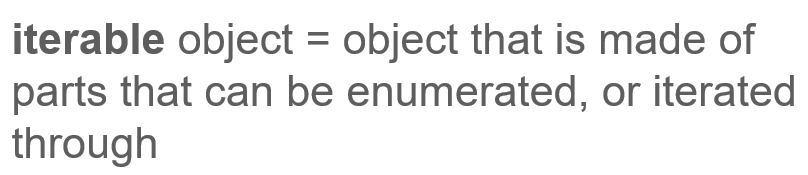An iterable object is any object that is made of parts that can be enumerated, or iterated.Let's start with a simple example. If you have a box full of chocolate truffles, you can enumerate the truffles. In other words, you can iterate through the contents of that box. One example of iteration, or enumeration, is the process of taking out one truffle at a time and counting it.Another example is a group of people. The group is made of individual people and we can enumerate them, or, to put it differently, we can iterate through the group.

So now, perhaps you're thinking, what does that have to do with loops?

Items as Iterable Objects

Well, let's play a game. Let's say we have our box of chocolate truffles again and for each truffle in the box, we take the truffle out, we think of a number between 1 and 10 and we ask our best friend to guess the number. If she or he guesses it, they get the truffle. If not, we eat the truffle. Chances are we'll probably eat most of the truffles, but that's ok.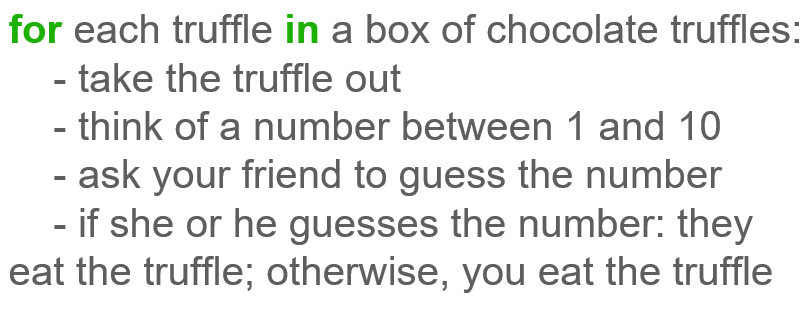Did you notice what we did here? We created a loop. The steps of taking one truffle out of the box, thinking of a number, asking our friend to guess - these are all steps that get repeated over and over until we run out of truffles. The condition that ends the loop is that we ran out of truffles, so unless you have access to infinite supplies of chocolate truffles, which would make me very jealous, you will eventually run out and the game will end. This is, of course, not an infinite loop.

Another thing to notice is that this loop is possible because we can iterate through the box of chocolate truffles. In other words, it's possible because we can enumerate the individual items that make up a larger object and perform some actions for each item.

Letters as Iterable Objects

It's very important that you understand this intuitively before we move to writing code examples that are more abstract, so let's go over another example.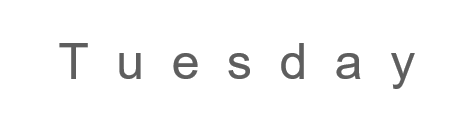Let's play another game. Let's take the current day of the week - let's say Tuesday. Not my favorite, but it will do for now.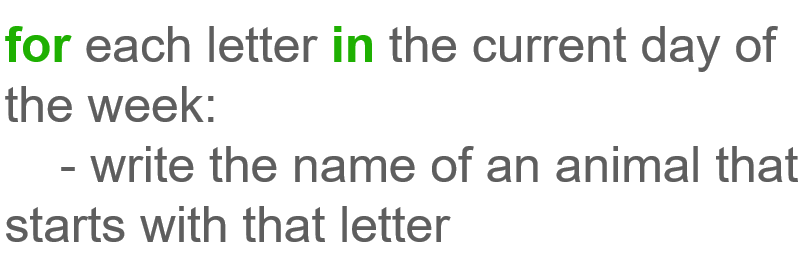For each letter in the current day, we want to write the name of an animal that starts with that letter.

How do we go about this? We start with the first letter and we try to think of an animal. Then, we move on to the next letter, etc. As we're doing this, we need to keep track of the current letter so we can write this down.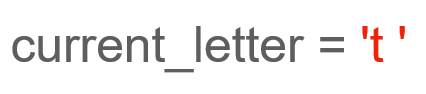Just for extra practice, I'm going to do this in Python syntax, so I'm going to say current_letter = 't'. Notice the quotes here, because 't' is a string. What's an animal that starts with t?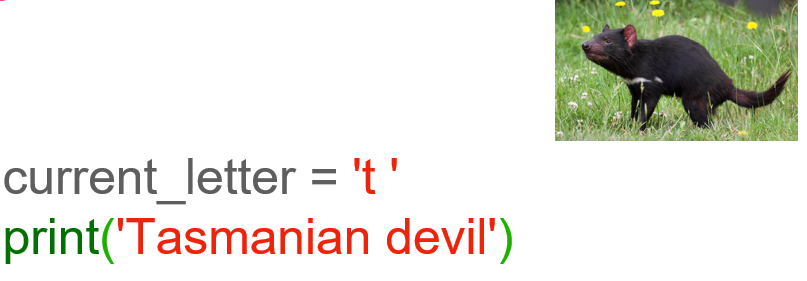Let's say Tasmanian devil. So we can print(Tasmanian devil)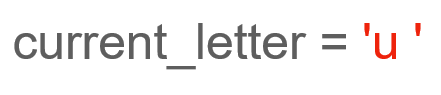Now we move to the next letter. That's u, so we can set current_letter = 'u'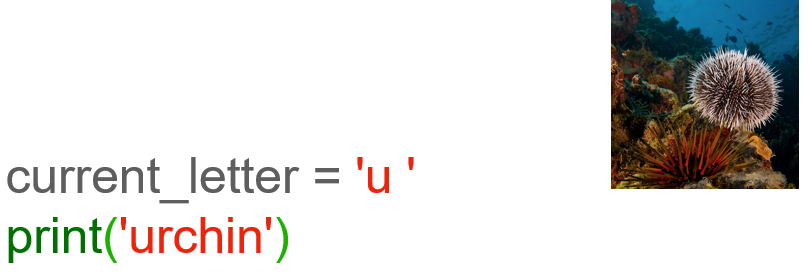The only one I can think of is urchin, so we print('urchin').

And so on, and so forth. I won't go over the remaining letters, but you get the point.

Iterable Objects in Python

To get back to our main point, iterable objects are objects that are made of parts or items that can be enumerated. In this case, we say that the object can be iterated through. Also, as we've just seen, iterable objects allow us to create loops, where conceptually, for each item that is part of the iterable object, we can perform some actions.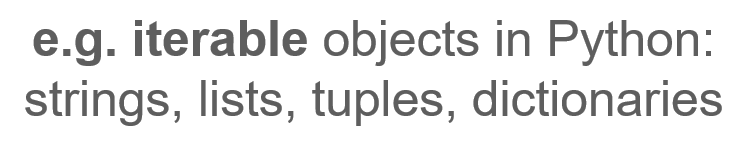So what are some iterable objects we learned about in Python? Well, for example, all the objects that are sequences are iterable. Strings are sequences of characters, so for each character in a string, we can perform some actions. Lists are ordered sequences of items, and for each item in a list, we can perform some actions. Tuples are also ordered sequences of items and again, for each item in a tuple, we can perform some actions. Same for dictionaries. Dictionaries are sequences of pairs consisting of a key and a matching value, and for each pair in a dictionary, we can again perform some actions.

Now that we've built some intuition around for-loops, we're ready for some coding. Let’s go over the anatomy of a for-loop in Python and explore some of its most common use cases.

For Loops

Let's explore the anatomy of a for loop in Python.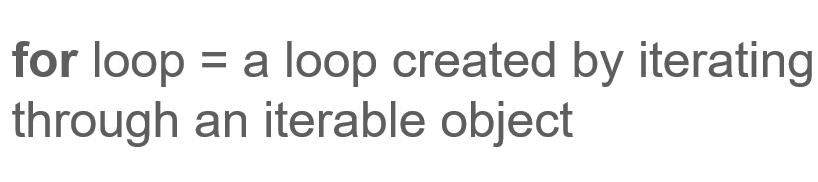A for loop is really just a type of loop in Python that's created by iterating through the items that make up an iterable object.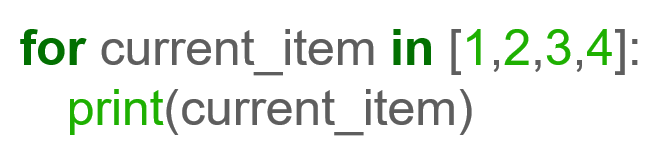In this example, we have a for loop that iterates through a list which contains the integers 1, 2, 3 and 4, and for each integer, it performs the action of printing that number.

Let's take this apart and look at the components that make up the loop.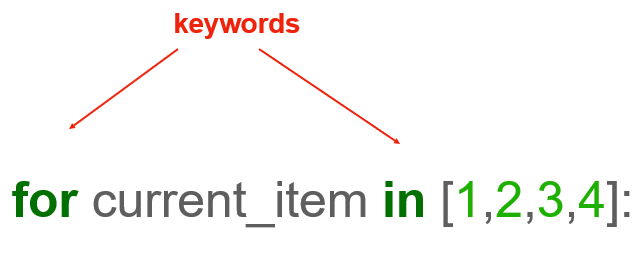First, notice the “for” and the “in” keywords highlighted in green. These are required to build the loop, and we naturally encountered them in our earlier examples.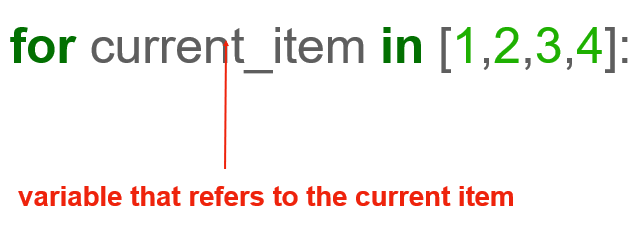One thing that often confuses beginners is that current_item variable there. That's a variable that refers to the current item, but you can name it whatever you want.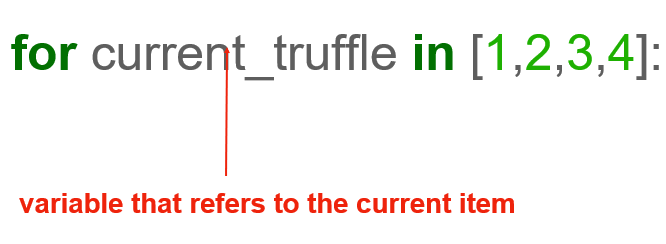For example, if we're talking about truffles in a chocolate box, we can call it current_truffle. As with all variable names, you should make it as descriptive as possible. This variable is automatically set by Python. So, Python performs the act of going through each item in the iterable object, which, in this case, is the list 1,2,3,4, and then, at each step, it stores the current item in that variable. This is important because in doing so, it makes that current item available to us.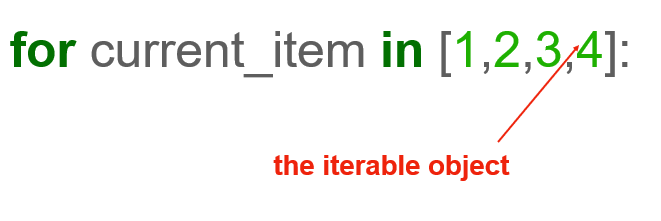Next, we have the iterable object. We already covered this in detail, so we won't go over it again, but this is just to tell you where in the for loop structure you need to place it.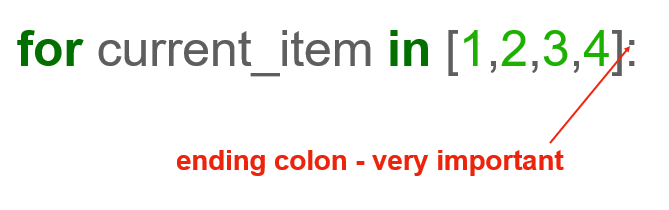And finally, on this line, we end with a colon, similar to how we did with if / elif / else statements. The colon is very important, so don't forget it.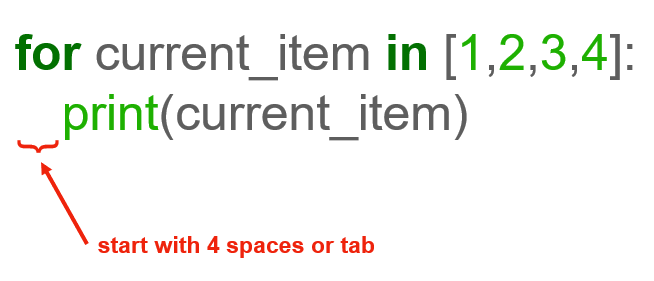Below, we have the set of lines of code that we want to execute for each item in the collection or sequence that we are iterating through. In this case, we're only doing a single thing. We're printing the current_item. So, if we run this code, it will first print 1, then it will print 2, then 3 and finally 4.

Notice the four spaces indentation. It can also be a tab, as I mentioned earlier. This, again, tells the Python interpreter which of the code is inside the loop vs outside the loop.

That's all for the anatomy of a Python for loop. Pretty simple, right?! Next, let’s dive into writing some code using for loops.

Writing For Loops in Python

Let's start by actually writing the code example we went over earlier: for current_item in [1,2,3,4]: print(current_item). If we run this code, we see that the numbers 1, 2, 3 and 4 get printed in order. Let's change this code to print something before the actual number. Let's say for current_item in [1,2,3,4]: print('current item is') print(current_item). We see that each of the two print lines gets executed for each of the integer numbers in the list.

# Let's create our for loop
for current_item in [1,2,3,4]:
print(current_item)
# Our output will be 1, 2, 3, 4

# If we change our code just a bit:
for current_item in [1,2,3,4]:
print('current item is')
print(current_item)
# Our output changes to include two lines:
#current item is, 1, current item is, 2, current item is, 3,
#current item is, 4

Just to drive home the fact that we can pick whatever name we want for the variable name here, let's change this to for boo in [1,2,3,4]: print(boo). And you see that, again, it prints all the numbers. So at each step of the loop, that variable gets updated to store the current item in our sequence. It starts by being 1, then it becomes 2 etc.

# Let's pick another name for our variable here
for boo in [1,2,3,4]
print(boo)
# Our output is 1, 2, 3, 4

Using Variables For Lists

Another thing I want to mention is that instead of the actual list, we can use a variable that stores a list. So let's say that we have a variable called truffles, and it stores the list [1,2,3,4,5]. We'll pretend that each truffle in the box has a number on it, so that's how we'd represent it in computer code. Say that we want to go over each truffle in the box, and just print out its number. We can just write for some_truffle in truffles: print(some_truffle). I named my current item some_truffle, but again, you can name it whatever is appropriate in each context. Note that, instead of the explicit list, we now use the name of the variable that stores the list, which in our case, is this truffles variable.

# Let's create our list:
truffles = [1,2,3,4,5]

# and our for loop
for some_truffle in truffles:
print(some_truffles)
# Our output will be 1, 2, 3, 4, 5

Also note that we don't actually have to use that current item in our logic. For example, we can just write truffles = [1,2,3,4,5] for some_truffle in truffles: print('Guess what number I have in mind!'). And you see that the result is that we print "Guess what number I have in mind!" 5 times. Why 5 times? Well, because this print statement is executed each time we pick a truffle from the box, and we have 5 truffles to pick.

# Let's use our list
truffles = [1,2,3,4,5]
# to create our for loop
for some_truffle in truffles:
print('Guess what number I have in mind!')
# Our output will be Guess what number I have in mind!, Guess what number I have in mind!,
#Guess what number I have in mind!, Guess what number I have in mind!,
#Guess what number I have in mind!

Now, you may say that we could achieve the same result by printing "Guess what number I have in mind!" 5 times by just writing 5 print statements, and that's correct. But there are two problems with that approach. The first is that, instead of 5, you might have 100 chocolate truffles, or perhaps even 1000. It is bad form, not to mention pretty tedious, to write a program that has 1000 repeated print lines. The second issue is that, if we read this list from a file, or as input provided by a user of our program, there's no way to know ahead of time how many items that list will have. So that's why for loops are essential. They allow us to traverse a sequence of items and execute some code for each item, regardless of how small or large that sequence is. As programmers, we don't have to think about it. We only need to worry about the part that gets repeated in each loop.

For Loops with Equations

Let's solve the following problem. Someone gives us a list of numbers and asks us to print the result of multiplying each item in that list. If they give us the list consisting of numbers 1,2,3, they want us to print 2, 4 and 6. How would we do that? Well, we don't yet know how to read input from users - we'll go over that in an upcoming article - but let's assume that somehow we read that list and let's say it consists of the numbers 1,2,3,4,5. So we can define a variable called input_nums short for input numbers, and let's set it to be the list [1,2,3,4,5]. Next, we write a for loop that goes over each element in that list, and outputs the result of multiplying it by 2. We do that by writing for num in input_nums: print(num*2). So here, again, this num variable will in turn take the values 1, then 2, then 3, then 4 and finally 5. And in each of the steps of the loop, we'll take that value, multiply it by 2, and print the result. If we run this code, we see that the output is 2,4,6,8,10, which is indeed what we wanted.

# Let's create our list
input_nums = [1,2,3,4,5]
# and create our for loop
for num in input_nums:
print(num * 2)
# Our output will be 2, 4, 6, 8, 10

Here's an even more interesting problem. Given a list of numbers, print only the ones that are multiples of 5. For example, 5 is a multiple of 5, so is 10, 15, 20, etc. So far, we have mostly been writing code for problems that we humans can do in our head, but this is starting to get into the realm of problems that, while we can still solve in our head, we can not do so nearly as fast as a computer.

So let's write code for how we'd solve this. First, let's consider the list of numbers we want to check, and let's say that the list is input_nums = [1,2,3,5,12,15,17,20]. We start by going over each item in that list, so for num in input_nums:. Now, what do we do next? First, we need to express the condition: if the number is a multiple of 5. That definitely sounds like an if statement, but how do we actually express "multiple of 5"? Remember the modulo operator? The one that is written as a percentage sign. This operator gives us the remainder of a division. A number is a multiple of 5 if, when dividing it by 5, we get the remainder 0. So we can write that as if num % 5 == 0:. This says if the remainder of dividing the current item in the list by 5 is 0, then let's print it: print(num). Let's run this code. And we get 5, 15, and 20 which indeed are the only numbers that are multiples of 5 in the list above.

# Let's define our list
input_nums = [1,2,3,5,12,15,17,20]

# Now let's create our for loop with our if statement
for num in input_nums:
if num % 5 == 0:
print(num)
# Our output is: 5, 15, 20

For Loops with Strings

Let's do another problem. Given a list of names, print the ones that begin with the letter a. Let's store this list in a variable, call it names, and let's set it to names = ['Andrea', 'Jim', 'Beyonce', 'Batman']. Totally random. So now, let's go over each name in this list: for name in names:. We want to only print the names that start with a. Perhaps you remember that, from strings, we can get the first character using its index position. So what we want to do is compare the character at index 0, which is the first character in the string, with A, and, if they are equal, we want to print the name. We do that by writing: if name == 'A': print(name). If we run this code, we get Andrea, which is indeed the only name that starts with an A in that list.

# Let's define our list
names = ['Andrea', 'Jim', 'Beyonce', 'Batman']

# Now let's write our code
for name in names:
if name == 'A':
print(name)
# Our output is: Andrea

Let's make this problem a bit more interesting. Let's say that, if the name does not start with an A, we just want to print the letter it starts with. That should be pretty easy. We take all the logic we have so far, and we add an else branch to our if. This else branch will only get executed if the condition on the if branch is not True. That is, it will only get executed if the name does not start with A. So, in this case, we just print(name) because name is the first character in the current name. Let's run this. And we get the full name Andrea, because it starts with an A, and then we get J for Jim, B for Beyonce and B for Batman because Jim, Beyonce and Batman don't start with an A, so we only output their first character.

# We'll keep our list
names = ['Andrea', 'Jim', 'Beyonce', 'Batman']

# Now, we'll update our code
for name in names:
if name == 'A':
print(name)
else:
print(name)
# Our output is Andrea, J, B, B

So you see, this is where we are starting to get more power, and are able to write more complex programs. I think this is where it becomes a matter of practice. The more code you write, the easier it will be for you to take a problem that is expressed in plain English and figure out what building blocks you need in order to express it in code. That ability only comes with practice, so the more you code, the easier it will be.

For Loops with Running Totals

Let’s go over some more hands-on practice with for loops and lists.

Let's say we have a stack of bills to pay, and we want to sum them up to figure out what our total bills for the month are. For simplicity, let's assume that we already have the amounts we owe for each bill, and we store those in a list variable. Let's call that bills_list and say that we have 70 dollars for internet, 100 for our phone bill, 1000 for rent - that last one can be either a really good deal or a really bad deal, depending on the city we're in, of course. And let's say we also spent about 600 dollars on food. Now, we want to sum those up to figure out how much we owe for those bare necessities of life.

Let's say we're going to store this total in a variable named total, and let's set that total to 0 initially. Next, we're going to go through each bill in our list of bills and add that bill to our running total. So we can write for bill in bills_list: total = the current total plus this bill, or total + bill. So now, once the whole for loop finishes, the variable named total should store the sum of all the bills in bills_list. We can print it, so print(total), and when we run this code we get 1770. That seems about right: 1000 + 100 + 600 + 70 is indeed 1770.

# Let's define our list and our variable
bills_total = [70, 100, 1000, 600]
total = 0

# and create our for loop
for bill in bills_list:
total = total + bill

print(total)
# Our output is: 1770

Let's go over this again and make sure it all makes sense. So, bills_list is just the list of bills. total is the variable where we store the final total amount. We set it to 0 because we need to define it and give it an initial value first before we can use it. That's because in Python, we cannot use a variable before it is defined. We set it to 0 because 0 is neutral to addition. You can add 0 to any number and it won't change the result. Next, the for loop should hopefully be pretty self-explanatory: we simply iterate over each of the bills in bills_list, and we use the variable named bill to refer to each of the bills in the list.

In the first step of the loop, this variable bill gets assigned the integer number 70, which is our first bill. Next, total, which is currently 0, gets added to 70, so total is now 70. So after the first step in the for loop, total is equal to 70.

On the second step of the loop, bill gets assigned the next value in the bills list, which is 100. Next, total, which on the previous step was updated to store the value 70, is added to 100 and we get 170. This new value is then assigned back to total. So after the second step, the value we store in total is now 170.

On the third step of the loop, the variable bill gets assigned the next value in the bills list, which is 1000. Next, total, which on the previous step was updated to store the value 170, is added to this new bill, which is 1000, and we get 1170. This new value is then assigned back to total. So after the third execution of the loop, the value we store in total is 1170.

Finally, on the fourth step of the loop, the variable bill gets assigned the last value in the bills list, which is 600. Next, total, which at this point is 1170, gets added to this bill, which is 600, and we get 1770. This new value is then assigned back to total. So after the fourth execution of the loop, the value we store in total is 1770.

At this point, the loop exits, which is another term we use to say that the loop ends. The next line in our program prints the current and final value stored in total, which is 1770.

Again, I want to stress how important the indentation is. Because the print function is not indented, it is not considered part of the for loop, so it won't run at each step of the loop. Instead it gets executed only once, when the loop ends. Compare that with this. In this case, print has the same indentation as total = total + bill. So what's going to happen? Well, the value we store in total is going to be updated as we discussed earlier, but in addition, this print statement is going to get run on every step of the loop. If we run this code, we get 70, 170, 1170 and 1770. That's because each time we update the value stored in total, we also print it. Those values match the ones we deduced earlier.

# Let's define our list and our variable
bills_total = [70, 100, 1000, 600]
total = 0

# and create our for loop
for bill in bills_list:
total = total + bill
# but this time, let's indent our print function
print(total)
# Our output is 70, 170, 1170, 1770

In your programs, you will often use both of these strategies. Sometimes you'll want to print the running total as it gets incremented. Sometimes you'll just want to print the total at the end, once everything was added up. Two fairly different outcomes separated by a simple indentation. So pay attention to those white spaces.

Next, we will go over the concept of a nested for loop, that is, a loop within a loop.

Nested For Loops

On this occasion, we'll also move on to iterating over other kinds of iterable objects. We mentioned, for example, that strings are also iterable because they are nothing but sequences of characters. So let's take a string, say name = 'James Bond'. Now, let's say we want to print each letter in the name three times. How do we do that? Well, whenever we hear words like “each”, we know a loop is needed.

So we start with for letter in name:. This variable named letter will be assigned, in turn, to each of the characters in the string 'James Bond'. So, how do we print a letter three times? Perhaps you remember that when we talked about strings, we mentioned that we can use the "*" operator, the same operator that is used for number multiplication. In this case, because the variable letter stores a string, it won't do number multiplication, but we can use it to print the letter string as many times as we want. So we just write here print(letter * 3). And if we run this, we get the result we want. We can replace 3 here with 5, and now we see each letter printed 5 times. Notice that even the space gets printed 5 times, but being a space, you can't see it. It's there though, trust me.

# Let's create our string
name = 'James Bond'

# and our for loop
for letter in name:
print(letter * 3)
# Our output is JJJ, aaa, mmm, eee,
#sss,    , BBB, ooo, nnn, ddd

Here's a more interesting problem. What if instead of a single name, we have a list of names, and, again, we want to print each letter of EACH of the name 3 times? This is a bit trickier, but bear with me. Let's start with the code we had before. First, instead of name, let's define a variable called names_list, and set it to the list ['James', 'Batman', 'Marie']. Now, if we go to the for loop and replace name with names_list and run it again, we get JamesJamesJames, followed by BatmanBatmanBatman, followed by MarieMarieMarie.

# Let's  try adjusting our string
names_list = ['James', 'Batman', 'Marie']
# and adjust our for loop
for letter in names_list:
print(letter * 3)
# Our output is JamesJamesJames,
#BatmanBatmanBatman, MarieMarieMarie

So not quite what we want. Remember, we want each letter printed 3 times for each of these names, not the names themselves printed three times. So, how do we do that?

Here's a silly way of doing it. We can just copy-paste the loop we had before and, instead of name, replace it in each instance with each of the names. So we end up having three loops that pretty much look the same, except that in each case we're iterating through a different name. For instance, this works: each letter in the name James is printed 3 times, and underneath each letter in the name Batman gets printed 3 times, and finally, each letter in the name Marie gets printed 3 times. What's the problem with it though?

# Let's use our same list
names_list = ['James', 'Batman', 'Marie']

#and create the 3 for loops
for letter in 'James':
print(letter * 3)

for letter in 'Batman':
print(letter * 3)

for letter in 'Marie':
print(letter * 3)
# Our output will be a long list: JJJ, aaa, mmm, eee, sss,
# BBB, aaa, ttt, mmm, aaa, nnn, MMM, aaa, rrr, iii, eee

Perhaps you're thinking I'm too lazy and I don't want to type that much. And that's actually a good instinct. When you program, in most cases, it pays off to write as little code as you can get away with. Less code written means less code that needs to be read, less code that needs to be updated, fewer opportunities for typos or other bugs. Besides, what if we had a list of 100 names instead of 3? I'm definitely not typing that.

So what's a different way to get this result? Well, look at these three for loops. What we're doing here is basically the same thing three times. We're just doing that thing to a different item. Doesn't that look like... a loop? If that was your guess, you are correct. Every time you repeat very similar steps like these, you can wrap what you're doing in a loop.

So we can solve this problem with a loop nested inside another loop. In the outer loop, we go over each of the names in our names_list and assign it, in turn, to a name variable. Then, inside that loop, we have another loop that goes over each letter in the name and prints it 3 times. So let's write that and see what it looks like. Let's delete these for loops and write for name in name_list: print(name). If we run this, we see that we print each name, as we'd expect. Now, instead of print(name), let's copy the loop we had before, so we replace it with for letter in name: print(letter * 3). Notice that the second for is indented 4 spaces or 1 tab character, just like the print statement before. Also, the print statement inside the second for loop is indented 4 spaces or 1 tab relative to the second “for” keyword.

# Let's keep our list
names_list = ['James',  'Batman', 'Marie']
# and adjust our for loop
for name in names_list:
for letter in name:
print(letter * 3)
# Now, our output is JJJ, aaa, mmm, eee, sss,
# BBB, aaa, ttt, mmm, aaa, nnn, MMM, aaa, rrr, iii, eee

This is crazy! We have a loop inside a loop. This whole second loop gets executed for EACH name in the names list. If we run this, we get what we want: each letter in the name James is printed 3 times, and underneath, each letter in the name Batman gets printed 3 times and finally, each letter in the name Marie gets printed 3 times.

You will often need to use these nested loops, so I wanted to show you an example of how you can use them. You can nest as many loops as you need, but I've rarely seen them nested more than 3 or 4 times because beyond that level of nesting, it becomes very hard to reason through them. However, this single nesting is very common, so definitely spend some time and make sure you understand it fully.

Nested For Loops with Nested Lists

Here's another very common use for nested loops. Let's say we have a list called nums_list, short for numbers list, and it consists of three nested lists. And each one of those nested lists has numbers inside it. So nums_list = [[1,2,3], [4,5,6], [7,8,9]]. We can certainly do this because lists are very flexible and can hold other lists inside them. So now, my tricky question is, how do we print each of the numbers we have here, on a separate line? Perhaps your first approach is to write something like for list_item in num_lists: print(list_item). However, if we run this, we see that the output is not what we want it to be. Why is that?

# Let's set our list
nums_list = [[1,2,3], [4,5,6], [7,8,9]]
# and create our for loop
for list_item in nums_list:
print(list_item)
# Our output is [1, 2, 3], [4, 5, 6], [7, 8, 9]

Well, the code does indeed do what we tell it to do. It goes over each item in the list and prints it out. But each of those items is itself a list, not a number. That's because nums_list has only 3 items: the list [1,2,3] is the first item, followed by a comma, followed by the list [4,5,6] which is the second item, followed by another comma, and finally, followed by the list [7,8,9] which is the third item. So Python looks at our code and says, ah, you want me to print the items in your nums_list, here they are. It's these three lists. And this can be a bit tricky perhaps to see at first: Python only looks at the top level when iterating through an object, it does not iterate INSIDE the nested objects by default.

But how do we get to the numbers inside the nested lists? It looks like for each one of those nested lists, we further need to iterate and print their own items. So we can change that code and write for list_item in nums_list: for num in list_item: print(num). Notice again the indentation. The second loop is inside the first loop, so we need the tab there (or 4 spaces if you don't like tab). If we run this code, we get what we want. How does that work though?

# We'll keep the same list
nums_list = [[1,2,3], [4,5,6], [7,8,9]]
# and use our nested for loop
for list_item in nums_list:
for num in list_item:
print(num)
# Our output here is 1, 2, 3, 4, 5, 6, 7, 8, 9

Well, we saw earlier that the variable list_item in turn gets assigned to the list [1,2,3], then the list [4,5,6] and finally the list [7,8,9] in the outer loop. Now, in the inner loop, we iterate over each one of these items and we print each of THEIR elements. So the outer loop begins by assigning the variable item_list to be the list [1,2,3], which is the first item in our nums_list. Then we execute the inner loop, and if we iterate over that, we get the integer 1, then the integer 2, then the integer 3, because those are the elements that are in it. Then, the inner loop ends and we're back in the outer loop. Now, item_list gets assigned the next value, which is the list [4,5,6] and then we again enter the inner loop and we iterate over each of the elements in [4,5,6]. When we print each of them, we get the integer 4, then the integer 5 and then the integer 6. And then the inner loop again ends and we're back in the outer loop. At this point, the outer loop assigns item_list to be the list [7,8,9], which is the last item in our nums_list. Finally, we enter the inner loop for the third time, and we iterate through the list [7,8,9] and print each item to finally get the integers 7, 8, and 9.

Play with these for a while. They might seem a bit difficult at times, and perhaps they remind you a bit of the movie Inception. It's indeed a bit like that. A loop inside a loop. As I said, it can get a bit more complex, and you can have a loop inside a loop inside a loop. We won't go over those examples right now, but the idea is exactly the same.

Now, we’ll look at how we can use for loops with two other Python iterables. Namely, tuples and dictionaries.

For Loops with Tuples

Let's talk about tuples. We won't go into as much detail with tuples, because everything that we talked about when we discussed lists or strings also applies to tuples. Let's just write an example. Let's say we have a tuple called coins_list = (1,5,10,25). So that's a tuple that stores the coin values that are in wide circulation in the United States. Let's quickly iterate through that list and, to make it more interesting, let's print out how many of each kind of coin we need to get to 1 dollar. So we can write for coin in coins_list: print(100/coin) print(coin). That works because 1 dollar is 100 cents, so, for example, if we want to know how many 5-cent coins we need to make a dollar, we just divide 100 by 5 and we'd get 20. So, if we run this code, we see that we need 100 1-cent coins to make a dollar, 20 5-cent coins, 10 10-cent coins, and finally, only 4 25-cent coins.

# Let's create our tuple
coins_list = (1, 5, 10, 25)

# Now let's create our for loop
for coin in coins_list:
print(100/coin)
print(coin)
# Our output is: 100.0, 1, 20.0, 5, 10.0, 10, 4.0, 25

For Loops with Dictionaries

Finally, let's iterate over some dictionaries also. Let's say we have a dictionary that gives us fruit prices. So market_prices = {'apples': 2.99, 'oranges': 3.99, 'avocados': 4.99}. Now, we can write: for item in market_prices: print(item). If we run this code, we get apples, oranges and avocados. Why is that? That's because, by default, when it comes to dictionaries, Python iterates through the list of keys, not through the list of pairs. It can be a bit confusing, but that's just how it works. If you want to iterate through the list of pairs, you need to change the loop to be for item in market_prices.items(): print(item). When we run this, we get the pairs indeed.

# Let's create our dictionary
market_prices = {'apples': 2.99, 'oranges': 3,99, 'avocados': 4.99}
# and create a for loop
for item in market_prices:
print(item)
# Our output is apples, oranges, avocados

# Let's adjust our for loop
for item in market_prices.items():
print(item)
# Now our output is: ('apples, 2.99), ('oranges', 3.99), ('avocados', 4.99)

We can also iterate through the list of values by changing the code to be for item in market_prices.values(): print(item). We learned about these functions item() and values() in earlier articles where we reviewed the dictionary data type.

# Let's keep our dictionary
market_prices = {'apples': 2.99, 'oranges': 3.99, 'avocados': 4.99}

# and adjust our loop to show the values
for item in market_prices.values():
print(item)
# Now our output is 2.99, 3.99, 4.99

I want to show you one more thing. You might think that it's a bit silly that, by default, iterating through Python dictionaries iterates through the keys, but it actually makes sense. Remember that, in a dictionary, we access values using their keys. So, let's go back to the first loop we wrote here, for item in market_prices: print(item). We run that again, and we see that it prints the keys. Well, how can you access the values that correspond to each of the key? Simple. Instead of print(item) here, we can write print(market_prices[item]). And if we run this, we see that we actually get the values. This works because in each step of the loop, this variable named item is assigned to some key in the dictionary, and, below, we use market_prices[item] to access the value that corresponds to that key.

# Our dictionary
market_prices = {'apples': 2.99, 'oranges': 3.99, 'avocados': 4.99}
# and our for loop that shows the keys
for item in market_prices:
print(item)
# This returns apples, oranges, avocados

# Another way to see our values
for item in market_prices:
print(market_prices[item])
# This will return 2.99, 3.99, 4.99

Remember, you need to be careful with dictionaries. Because they are unsorted sequences, the order in which you get the keys is not guaranteed. But you'll definitely get all of them.

While we can use for loops with just about every type of iterable in Python, they are most commonly used with lists, and particularly with lists of numbers. Now, let’s explore a more convenient way to generate a list of numbers: the range function.

For Loops and the Range Function

We'll talk more about functions in a bit, so I won't go into too much detail about what a function is just now. Instead, I just want to show you how you can use range with loops. Primarily, let's consider this use case. I want to write the text hello 5 times. How do I do this? Well, as we've seen before, we could just write print('hello') five times, but that would be silly because we actually know how to use loops. So instead, we can write this: for i in [0,1,2,3,4]: print('hello'). And this works. If I run it, I see the word hello printed 5 times. I started counting at 0 here, but there are 5 elements in that list and the word hello is printed for each of them, so it's printed 5 times.

# Let's create our for loop
for i in [0,1,2,3,4]:
print('hello')
# Our output is hello, hello, hello, hello, hello

Ok, but what if I now have to print the word hello 10 times or 100 times? I guess I could just write all the numbers from 0 to 9, or to 99, but there has to be a better way to do that. And indeed there is. We can just use the range function like so: for i in range(9): print('hello'). If we run this, we see that the word hello was printed 9 times. How does that work? Well, the range function returns a list of numbers - in our case, it generates 9 numbers, starting at 0 and ending at 8. We can also replace our earlier code with: for i in range(5): print('hello'). And if we run it again, we see the word hello printed 5 times. There are more advanced uses of the range function too, but for now, remember that you can just use it like this, to repeat an action a number of times.

# Let's use the range function
for i in range(9):
print('hello')
# Our output here is hello, hello, hello, hello,
#hello, hello, hello, hello, hello

# Let's try it again
for i in range(5):
print('hello')
# And now we get hello, hello, hello, hello, hello

This concludes our foray into Python for loops. It's quite a bit of material if you're new to it, so take your time, re-read these segments if you need to, and practice, practice, practice, to get comfortable with loops. Now that we know if statements and for loops, and we covered the main data types in Python, we can write programs that solve much more complex problems, as we've seen.Ciprian Stratulat

Ciprian is a software engineer and the CTO of Edlitera. As an instructor, Ciprian is a big believer in first building an intuition about a new topic, and then mastering it through guided deliberate practice.

Before Edlitera, Ciprian worked as a Software Engineer in finance, biotech, genomics and e-book publishing. Ciprian holds a degree in Computer Science from Harvard University.